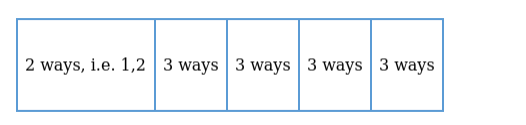# How many 5-digit numbers can be formed by using the digits 0, 1 and 2?

Question:

How many 5-digit numbers can be formed by using the digits 0, 1 and 2?

Solution:

Given: We have 3 digits, i.e. 0, 1 and 2

To Find: Number of 5-digit numbers formed

Let us represent the arrangementFor forming a 5-digit number, we have to fill 5 vacant spaces.

But the first place cannot be filled with 0 , hence for filling first place, we have only 1 and 2

First place can be filled with 2 numbers, i.e. $1,2=2$ ways

Second place can be filled with 3 numbers $=3$ ways

Third place can be filled with 3 numbers $=3$ ways

The fourth place can be filled with 3 numbers $=3$ ways

The fifth place can be filled with 3 numbers $=3$ ways

Total number of ways $=2 \times 3 \times 3 \times 3 \times 3=162$

1625 -digit numbers can be formed by using the digits 0,1 and 2 .